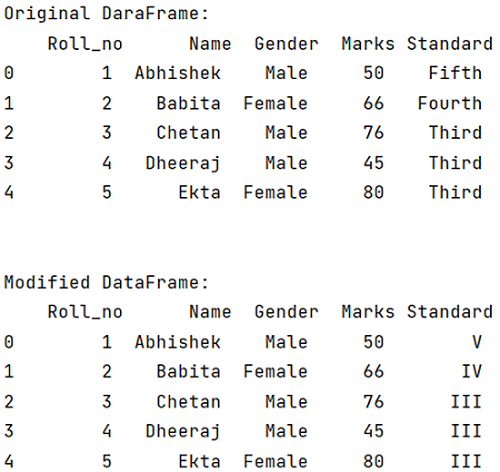# How to remap values in pandas using dictionaries?

Learn how to remap values in pandas using dictionaries? By Pranit Sharma Last updated : September 19, 2023

Pandas is a special tool which allows us to perform complex manipulations of data effectively and efficiently. Inside pandas, we mostly deal with a dataset in the form of DataFrame. DataFrames are 2-dimensional data structure in pandas. DataFrames consists of rows, columns and the data. DataFrame can be created with the help of python dictionaries or lists but in real world, csv files are imported and then converted into DataFrames.

## Problem statement

After converting CSV files into DataFrame we perform various operations on this DataFrame to get the desired output. Sometimes we need to remap all the values of a column in the DataFrame. Here, we are going to learn how to remap the column of a value in DataFrame

## Remapping values in pandas using dictionaries

The DataFrame.replace() method is used to replace the target value with the desired value. This method searches all the appearances of the target value and replaces all the appearances with the desired value. The target value and desired value can be passed in the form of key and value of a dictionary.

Syntax:

```DataFrame.replace(
to_replace=None,
value=NoDefault.no_default,
inplace=False,
limit=None,
regex=False,
method=NoDefault.no_default
)
```

Let us understand with the help of an example:

## Python program to remap values in pandas using dictionaries

```# Importing pandas package
import pandas as pd

# Creating a dictionary
d = {
'Roll_no': [ 1,2,3,4,5],
'Name': ['Abhishek', 'Babita','Chetan','Dheeraj','Ekta'],
'Gender': ['Male','Female','Male','Male','Female'],
'Marks': [50,66,76,45,80],
'Standard': ['Fifth','Fourth','Third','Third','Third']
}

# Creating a DataFrame
df = pd.DataFrame(d)

# Display original DataFrame
print("Original DataFrame:\n",df,"\n\n")

# Setting a target value to replace Standard column
dict = {'Fifth':'V','Fourth':'IV','Third':'III'}

# Remapping the values of the column standard
result = df.replace({'Standard':dict})

# Display Modified DataFrame
print("Modified DataFrame:\n",result,'\n')
```

### Output

The output of the above program is: